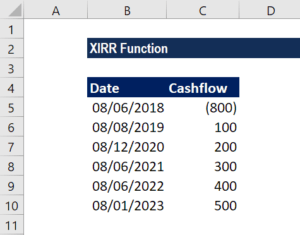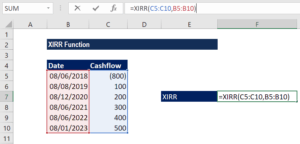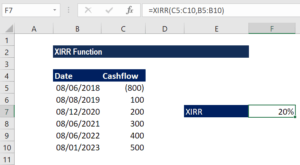# XIRR Function

Get the Internal Rate of Return (IRR) for a series of cash flows that may not be periodic

## What is the XIRR Function?

The XIRR function is categorized under Excel financial functions. It will calculate the Internal Rate of Return (IRR) for a series of cash flows that may not be periodic. It does this by assigning specific dates to each individual cash flow. The main benefit of using the XIRR Excel function is that such unevenly timed cash flows can be accurately modeled. To learn more, read why to always use XIRR over IRR in Excel modeling.

In financial modeling, the XIRR function is useful in determining the value of an investment or understanding the feasibility of a project that does not have regularly periodic cash flows. It helps us understand the rate of return earned on an investment. Hence, it is commonly used in evaluating and choosing between two or more investments.

### Formula

=XIRR(values, dates,[guess])

The formula uses the following arguments:

1. Values (required argument) – This is the array of values that represent the series of cash flows. Instead of an array, it can be a reference to a range of cells containing values.
2. Dates (required argument) – This is a series of dates that correspond to the given values. Subsequent dates should be later than the first date, as the first date is the start date and subsequent dates are future dates of outgoing payments or income.
3. [guess] (optional argument) – This is an initial guess – or estimate – of what the IRR will be. If omitted, Excel takes the default value of 10%.

Excel uses an iterative technique for calculating XIRR. Using a changing rate (starting with [guess]), XIRR cycles through the calculation until the result is accurate within 0.000001%.

### How to use the XIRR Function in Excel?

To understand the uses of the XIRR function, let’s consider a few examples:

#### XIRR Example

Suppose a project started on January 1, 2018. The project gives us cash flows in the middle of the first year, after 6 months, then at the end of 1.5 years, 2 years, 3.5 years, and annually thereafter. The data given is shown below:The formula to use will be:We will leave the guess as blank so Excel takes the default value of 10%.

We get the result below:### Things to remember about the XIRR Function

1. Numbers in dates are truncated to integers.
2. XNPV and XIRR are closely related. The rate of return calculated by XIRR is the interest rate corresponding to XNPV = 0.
3. Dates should be entered as references to cells containing dates or values returned from Excel formulas.
4. #NUM! error – Occurs if either:
1. The values and dates arrays are of different lengths;
2. The given arrays do not contain at least one negative and at least one positive value;
3. Any of the given dates precede the first date provided;
4. The calculation fails to converge after 100 iterations.
5. #VALUE! error – Occurs when either of the dates given cannot be recognized by Excel as valid dates.

Thanks for reading CFI’s guide to the Excel XIRR function. By taking the time to learn and master these functions, you’ll significantly speed up your financial analysis. To learn more, check out these additional CFI resources:

• Excel Functions for Finance